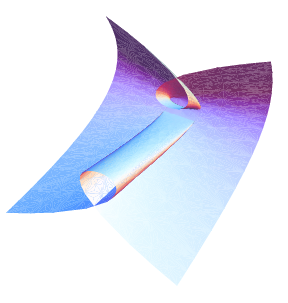Introduction to Algebraic Geometrypcmi2008David Perkinson
Department of Mathematics
Reed College, Portland OR

Course Description
Algebraic geometry is the study of solutions to systems of polynomial equations. It is a central and very active area of modern mathematics with deep connections to commutative algebra, complex analysis, number theory, combinatorics, and topology. It has applications in physics, robotics, coding theory, optimization theory, and more recently in biology and statistics. We will begin by developing the standard "dictionary" for translating between algebraic properties of polynomials and geometric properties of their solution sets. Special topics include Hilbert functions and resolutions of ideals, enumerative geometry, Grassmannians and the Schubert calculus, and computational aspects involving Gröbner bases.

What can I do to prepare?
All students should visit this algebra review page. I am assuming everyone has had a good course in linear algebra. If you have not had a course in abstract algebra or if your algebra is weak, please make sure to read the algebra review sheet on that page and do the exercises. I will be assuming the ring theory presented there.

Schedule
There will be 15 weekday morning lectures and afternoon problem sessions. The lecture titles are in the table below.

 1 The Dictionary 2 Nullstellensatz, Noetherian 3 Lectures lecture     problem set lecture    problem set lecture    problem set lecture    problem set lecture    problem set lecture    problem set lecture    problem set lecture    problem set *CoCoA handout* module The ultimate free resolution An overview of the multiplicity conjecture, and a proof lecture    problem set lecture    problem set lecture    problem set lecture    problem set An introduction to the Schubert calculus. lecture    problem set Macaulay An introduction by Buchberger. lecture    problem set lecture